# Light Reflection and Refraction Class 10 Important Questions Science Chapter 10

Solved the very best collection of Light Reflection and Refraction Class 10 Science Important Questions and Answers Chapter 10 Pdf from the latest NCERT edition books, It will help you in scoring more marks in CBSE Exams.

## Light Reflection and Refraction Class 10 Important Questions with Answers Science Chapter 10

### Light Reflection and Refraction Class 10 Important Questions Short Answer Type

Question 1.
Draw the following diagram in your answer book and show the formation of image of the object AB with the help of suitable rays.   (CBSE 2008)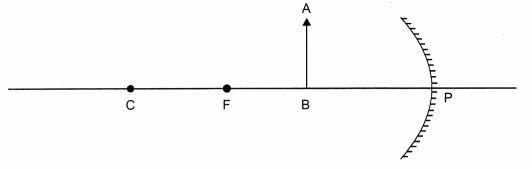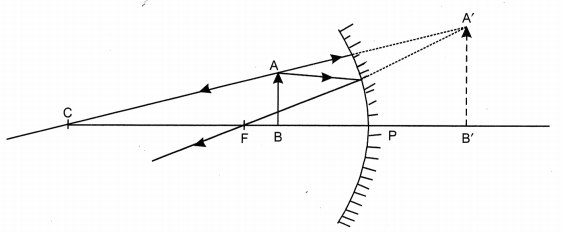Question 2.
Draw ray diagrams to represent the nature, position and relative size of the image formed by a convex lens for the object placed:
(a) At 2F
(b) Between F1 and the optical center O of lens   (CBSE 2008)
(a)(b)Question 3.
Which kind of mirrors are used in the headlights of a motor-car and why?   (CBSE 2008)
Concave mirror, to get the parallel beam of light.

Question 4.
(a) It is desired to obtain an erect image of an object using a concave mirror of focal length 20 cm.
(i) What should be the range of distance of the object from the mirror?
(ii) Will the image be bigger or smaller than the object?
(iii) Draw a ray diagram to show the image formation in this case.
(b) One-half of a convex lens of focal length 20 cm is covered with a black paper.
(i) Will the lens produce a complete image of the object?
(ii) Show the formation of image of an object placed at 2F1 of such covered lens with the help of a ray diagram.
(iii) How will the intensity of the image formed by half covered lens compare with non-covered lens? (CBSE 2008)
(a) (i) Range of the object distance is 0 to 20 cm from the pole.
(ii) Image will be bigger than the object.
(iii) Ray diagram: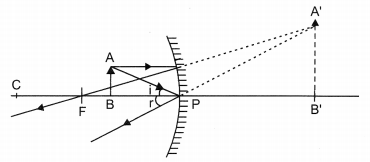(b) (i) Yes, complete image will be formed.
(ii)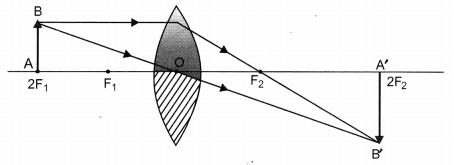(iii) Intensity will be reduced as the light falling on the lower (covered) portion will not reach the position of image.

Question 5.
Which type of mirrors are used to give an erect and enlarged image of an object?   (CBSE 2008)
Concave mirror.

Question 6.
If a light ray IM is incident on the surface AB as shown, identify the correct emergent ray.   (CBSE 2008)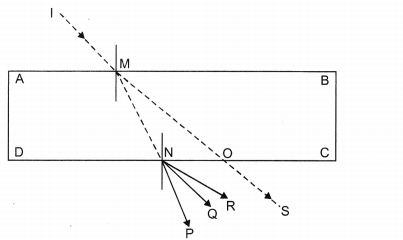Q as it has to be parallel to S.

Question 7.
An object of 2 cm high is placed at a distance of 64 cm from a white screen on placing a convex lens at a distance of 32 cm from the object it is found that a distant image of the object is formed on the screen. What is the focal length of the convex lens and size of the image formed on the screen? Draw a ray diagram to show the formation of the image in this position of the object with respect to the lens.   (CBSE 2008)
Since the object-screen distance is double of object-lens separation, the object is at a
distance of 2 from lens and the image should be of the same size of the object.So 2f = 32 ⇒ f = 16 cm
Height of image = Height of object = 2 cm

Question 8.
The power of a lens is -4.0 D. What is the nature of this lens? (CBSE 2008)
Negative power is associated with only concave lens.

Question 9.
Redraw the given diagram and show the path of refracted ray.   (CBSE 2008)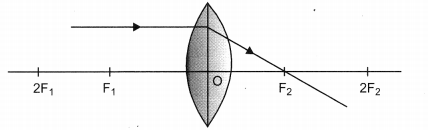Question 10.
A convex lens has a focal length of 10 cm. At what distance from the lens should the object be placed so that it gives a real and inverted image 20 cm away from the lens? What would be the size of the image formed if the object is 2 cm high? With the help of a ray diagram show the formation of the image by the lens in this case.   (CBSE 2008)
f = +10 cm, v = +20 cm as image is real and inverted. Height of the object = 2 cm (say +ve)
Using $$\frac{1}{f}=\frac{1}{v}-\frac{1}{u}$$, we get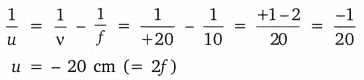Image will be of the same size as that of object (as u = v) and hence, the height of the image will be 2 cm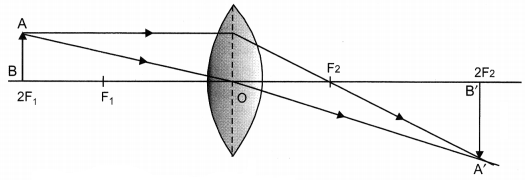Question 11.
The speed of light in air is 3.0 + 108 m/s
The speed of light in a transparant liquid is 2.0 + 108 m/s
A ray of light is incident on the surface of the liquid at an angle of incidence of 40°.
Calculate
(i) the refractive index of the liquid.
(ii) the angle of refraction in the liquid.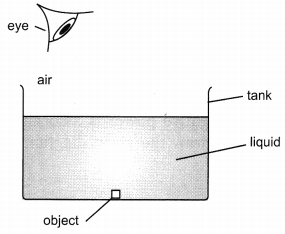(iii) Draw two rays in the above diagram to show (how light reaches our eye from the object placed in water).
(i) n = speed of light in air/speed of light in liquid
n = 3 × 108/2.0 × 108
n = 1.5
(ii) n = sin I / sin r ⇒ sin r = $$\frac{\sin i}{n}$$
sin r = sin 40° / 1.5 (which is 25°)
(iii)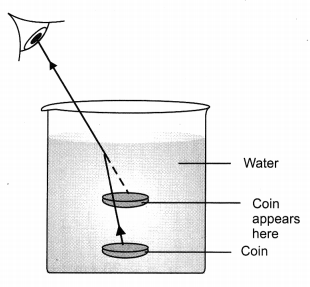Question 12.
Define and show on a diagram, the following terms relating to a concave mirror:
(i) Aperture
(ii) Radius of curvature   (Foreign 2011)
(i) The diameter of the reflecting surface of the mirror is called aperture.
(ii) The radius of the sphere of which the reflecting surface of the spherical mirror forms a part is called the radius of curvature of the mirror.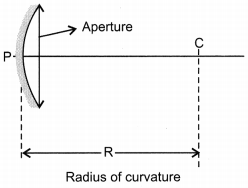Question 13.
Define the focus of a concave mirror. If the radius of curvature of a convex mirror is 30 cm, what would be its focal length?   (CBSE 2011)
The point on the principal axis where all the rays parallel to it meets is called the focus.
If R = 30 cm, then f = $$\frac{\mathrm{R}}{2}$$; f = 30 cm/2 = 15 cm.
Focal length is 15cm.

Question 14.
Distinguish between a real and a virtual image of an object. What type of image is formed (i) by a plane mirror, (ii) on a cinema screen? (CBSE 2011)
Real image:

1. The image is formed on the screen
2. The image is always inverted The image is always erect

Virtual image:

1. The image is not formed on the screen.
2. The image is always erect

Plane mirror always forms a virtual image and on the cinema screen real image is formed.

Question 15.
Draw a ray diagram and also state the position, the relative size and the nature of image formed by a concave mirror when the object is placed at the centre of curvature of the mirror.   (CBSE 2011)Position of object: At C
Position of image: At C
Nature of image: Real, inverted and of same size.

Question 16.
Define ‘refractive index of a transparent medium’. What is its unit? Which has a higher refractive index, glass or water?   (CBSE 2011)
The ratio of the speed of light in the vacuum (c) to the speed of light in the given medium (v) is called refractive index.
n = c/v
It has no unit as it is the ratio.
The refractive index of glass is more than water as it is optically denser.

Question 17.
A ray of light travelling in air enters obliquely into water. Does the light ray bend towards or away from the normal? Why? Draw a ray diagram to show the refraction of light in this situation.   (CBSE 2011)Light bends towards the Normal as the light is passing from rare medium i.e. air to the denser medium i.e. water. The ray of light travels with the lesser speed in the denser medium and hence bends.

Question 18.
(a) “The refractive index of diamond is 2.42”. What is the meaning of this statement?
(b) Name a liquid whose mass density is less than that of water but it is optically denser than water.   (CBSE 2011)
(a) This means that the ratio of speed of light in air and the speed of light in diamond is equal to 2.42
(b) Kerosene.
As kerosene floats on water but its optical density is higher than water.

Question 19.
An object is placed between infinity and the pole of a convex mirror. Draw a ray diagram and also state the position, the relative size and the nature of the image formed.   (CBSE 2011)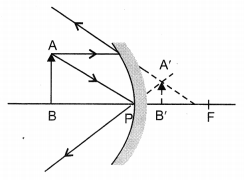Position of the object: Between infinity and P of the mirror.
Position of image: Between P and F, behind the mirror
Nature of image: Virtual, erect and diminished

Question 20.
What is understood by lateral displacement of light? Illustrate it with the help of a diagram. List any two factors on which the lateral displacement of a particular substance depends.   (CBSE 2011)
Lateral displacement is the perpendicular distance between the incident ray produced and the emergent ray. Lateral displacement in the diagram is BL. The lateral displacement depends on the thickness of the slab, the incident and refraction angles.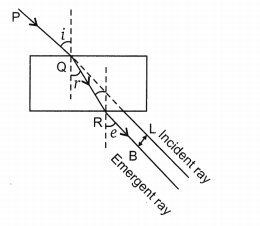Question 21.
Draw the ray diagram and also state the position, relative image formed by a concave mirror when the object is placed between its centre of curvature, C and focus, F.   (CBSE 2011)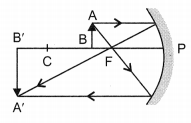Position of object: Between F and C
Position of image: Beyond C
Nature of image: Real, inverted and enlarged.

Question 22.
List four properties of the image formed by a plane mirror.   (CBSE 2012)
Properties of image formed by a plane mirror:

1. It is always virtual and erect.
2. Its size is equal to that of the object.
3. It is formed at the same distance behind the mirror as the object is in front of the mirror.
4. It is laterally inverted.

Question 23.
List four properties of the image formed by a convex mirror.   (CBSE 2012)
Properties of image formed by a convex mirror:

1. It is always formed behind the mirror, between the pole and its focus
2. It is always virtual and erect.
3. Its size is always smaller than the object.
4. Magnification is always positive.

Question 24.
List four properties of the image formed by a concave mirror, when object is placed between focus and pole of the mirror.   (CBSE 2012)

1. The image is formed behind the mirror
2. It is enlarged i.e., magnified.
3. It is virtual
4. It is erect.

Question 25.
To instruct a ray diagram, we use two light rays which are so chosen that it is easy to know their directions after refraction from the lens. List these two rays and state the path of these rays after refraction. Use these two rays to locate the image of an object placed between F and 2F of a convex lens. (  CBSE 2012)
Two rays choose for refraction:

1. A ray of light parallel to the principal axis.
2. A ray of light passing through the optical centre of a lens.

Path of these rays after refraction:
In case of convex lens, the first ray will pass through the principal focus on the other side of the lens. In case of concave lens, the first ray will

1. appear to diverge of
2. appear to come from the principal focus positioned on the same side of the object.

The second ray emerged from the lens without any deviation in the path.Question 26.
State the type of mirror preferred as (i) rear view mirror in vehicles, shaving mirror.
In rear view vehicles the mirror used is convex and in the shaving mirrors it is concave. Convex mirror is used as a rear view mirror because it gives the wider view of the traffic behind and the image obtained is erect.

The shaving mirror is concave because the image formed is highly magnified and virtual as the object is placed between the F and the P of the mirror.

Question 27.
The image of a candle flame placed at a distance of 45 cm from a spherical lens is formed on a screen placed at a distance of 90 cm from the lens.
Identify the type of lens and calculate its focal length. If the height of the flame is 2 cm, find the height of its image. (CBSE 2012)
Nature of spherical lens = convex lens
Given: u = – 45 cm, v = + 90 cm, h1 = + 2 cm
Using lens formula,f = + 30 cm
focal length of convex lens is 30 cm.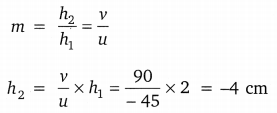The image is formed below the principal axis and is 4 cm in height.

Question 28.
A 4 cm tall object is placed perpendicular to the principal axis of a convex lens of focal length 24 cm. The distance of the object from the lens is 16 cm. Find the position, size and nature of the image formed, using the lens formula. (CBSE 2012)
For convex lens
f = + 24 cm, u = – 16 cm
lens formula,
$$\frac{1}{f}=\frac{1}{v}-\frac{1}{u}$$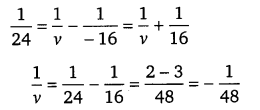v = – 48 cm
h1 = 4 cm
magnification,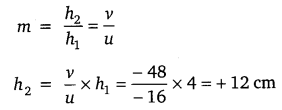Position of the image is 48 cm away from the mirror on the same side of the object as it is negative sign.
Height of image is 12 cm, 3 times the size of the object and the image lies above the principal axis and is virtual and erect as the sign is positive.

Question 29.
Name the type of mirror used in the following situations:
(ii) Rear-view mirror of vehicles
(i) Headlight of car: Concave mirror, it is used as a reflector and is placed behind the bulb such that, when the bulb illuminates the parallel beam of light illuminates the road.

(ii) Rear view mirror: Convex mirror is used as a rear view mirror because it gives the wider view of the traffic behind and th6 image obtained is erect.

(iii) Solar furnace: Concave mirror is used in the solar furnace because it is a converging mirror and can reflect all the rays at focus. The temperature at focus increases at that point.

Question 30.
A 6 cm tall object is placed perpendicular to the principal axis of a convex lens of focal length 15 cm. The distance of the object from the lens is 10 cm. Find the position, size and nature of the image formed, using the lens formula (CBSE 2012)
For convex lens f = + 15 cm, u = – 10 cmv = – 48 cm
h1 = 4 cm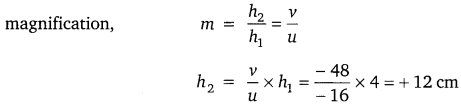Position of the image is 48 cm away from the mirror on the same side of the object as it is negative sign.
Height of image is 12 cm, 3 times the size of the object and the image lies above the principal axis and is virtual and erect as the sign is positive.

Question 29.
Name the type of mirror used in the following situations:
(ii) Rear-view mirror of vehicles
(i) Headlight of car: Concave mirror, it is used as a reflector and is placed behind the bulb such that when the bulb illuminates the parallel beam of light illuminates the road.

(ii) Rear view mirror: Convex mirror is used as a rear view mirror because it gives the wider view of the traffic behind and the image obtained is erect.

(iii) Solar furnace: Concave mirror is used in the solar furnace because it is a converging mirror and can reflect all the rays at focus. The temperature at focus increases at that point.

Question 30.
A 6 cm tall object is placed perpendicular to the principal axis of a convex lens of focal length 15 cm. The distance of the object from the lens is 10 cm. Find the position, size and nature of the image formed, using the lens formula (CBSE 2012)
For convex lens f = + 15 cm, u = – 10 cm
Lens formula,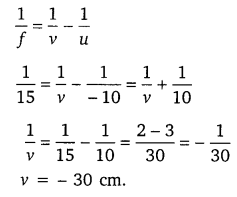The image is formed on the same side of the object at the distance of 30cm. Negative sign indicates that the image is virtual.The size of the object was 6 cm and the size of the image is 18cm. The positive sign indicates that image is erect.

Question 31.
List four characteristics of the images formed by plane mirrors.   (Delhi 2015)

1. Virtual
2. Erect
3. Same size as the object
4. As far behind the mirror as the object is in front of it.
5. Laterally inverted.

Question 32.
To construct a ray diagram we use two rays of light which are so chosen that it is easy to determine their directions after reflection from the mirror. Choose these two rays and state the path of these rays after reflection from a concave mirror. Use these two rays to find the nature and position of the image of an object placed at a distance of 15 cm from a concave mirror of focal length 10 cm.   (Delhi 2015)The candidate may choose any two of the following rays:

1. A ray parallel to the principal axis, after reflection, will pass through the principal focus of a concave mirror.
2. A ray passing through the principal focus of a concave mirror after reflection will emerge parallel to the principal axis.
3. A ray passing through the centre of curvature of a concave mirror after reflection is reflected back along the same path.
4. A ray incident obliquely to the principal axis towards the pole of a concave mirror is reflected obliquely, making equal angles with the principal axis.

Question 33.
The absolute refractive indices of glass and water are 4/3 and 3/2 respectively. If the speed of light in glass is 2 x 108 m/s, calculate the speed of light in
(i) vacuum,
(ii) water.   (AI 2015)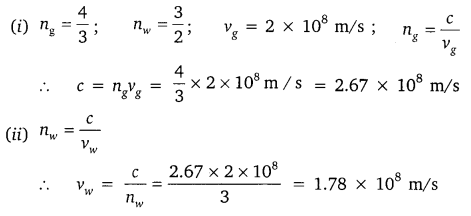Question 34.
If the image formed by a mirror for all positions of the object placed in front of it is always erect and diminished, what type of mirror is it? Draw a ray diagram to justify your answer. Where and why do we generally use this type of mirror?   (AI 2015)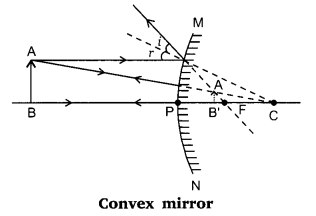Uses: It is used as rear view mirror in vehicles. It is also used in Malls, Hotels, Airports for security reasons.
Reason:

• It forms erect image.
• It gives wider field of view

Question 35.
Draw a ray diagram to show the path of light when it travels through glass slab.   (CBSE 2015)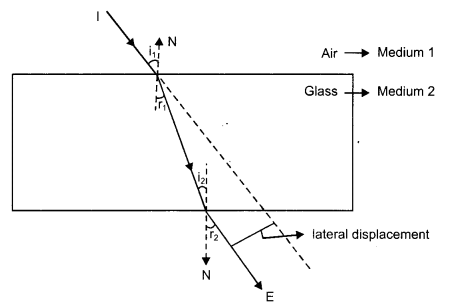Incident ray I enters the glass slab forms an angle of incidence ‘i’. Its bends towards the normal and forms an angle of refraction ‘r’.
The emergent ray is parallel to the incident ray.

Question 36.
Draw a ray diagram to show the path of the reflected ray in each of the following cases. A ray of light incident on a convex mirror (Foreign 2015)
(a) strikes at its pole making an angle from the principal axis.
(b) is directed towards its principal focus.
(c) is parallel to its principal axis.
(a)(b)(c)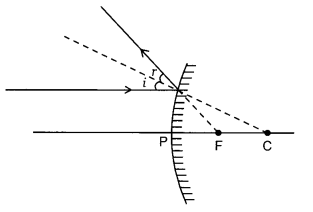Question 37.
State two positions in which a concave mirror produces a magnified image of a given object. List two differences between the two images.   (Delhi 2016)

1. When the object is placed in front of the mirror-
(i) between its pole and focus
(ii) between the focus and infinity
2. In case (i) the image is virtual and erect.
3. In case (ii) the image is real and inverted.

Question 38.
Draw the following diagram, in which a ray of light is incident on a concave/convex mirror, on your answer sheet. Show the path of this ray, after reflection, in each case.   (Delhi 2016)Question 39.
AB and CD, two spherical mirrors, form parts of a hollow spherical ball with its centre at O as shown in diagram. If arc AB = $$\frac{1}{2}$$ arc CD, what is the ratio of their focal lengths? State which of the two mirrors will always form virtual image of an object placed in front of it why?   (Foreign 2016)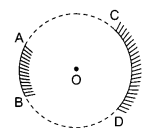Focal length of both the mirrors will be the same / 1: 1.
Mirror AB will always form virtual image as it is a diverging / convex mirror.

Question 40.
If the image formed by a lens for all positions of the object placed in front of it is always virtual, erect and diminished, state the type of the lens. Draw a ray diagram in support of your answer. If the numerical value of focal length of such a lens is 20 cm, find its power in new cartesian sign conventions.   (Foreign 2016)
Diverging lens / concave lens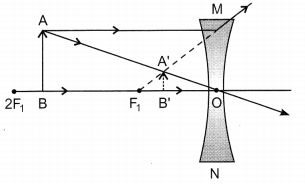Focal length = -20 cm (lens is concave, hence f is -ve)
Power = P = 1/f = 100/ -20 cm = -5D.

Question 41
The image of an object formed by a mirror is real, inverted and is of magnification – 1. If the image is at a distance of 40 cm from the mirror, where is the object placed? Where would the image be if the object is moved 20 cm towards the mirror? State the reason and also draw ray diagram for the new position of the object to justify your answer.  (AI 2016)
For magnification of 1, the object and image must be at centre of curvature Object distance = 40 cm.When object is moved 20 cm towards the mirror, position of the image is at infinity.
Reason – Focal length of the mirror = 20 cm.
If the object is moved 20 cm towards the mirror then its new position would be at the focus of the mirror.

### Light Reflection and Refraction Class 10 Important Questions Long Answer Type

Question 1.
(a) If the image formed by a lens is diminished in size and erect, what type of lens is it?
(b) Name the point on the lens through which a ray of light passes undeviated.
(c) An object is placed perpendicular to the principal axis of a convex lens of focal length 20 cm. The distance of the object from the lens is 30 cm.
Find (i) the position (ii) the magnification and (iii) the nature of the image formed.   (CBSE 2011)
(a) Concave lens
(b) Optical centre
(c) u = – 30 cm, f = 20 cm
(i) $$\frac{1}{f}=\frac{1}{v}-\frac{1}{u}$$v = 60 cm

Image is formed at a distance of 60 cm from the lens on the right side.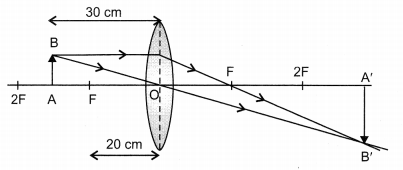(ii) m = $$\frac{v}{u}=\frac{60}{-30}$$ = -2
image is inverted and double the size of the object.
(iii) Image is real, inverted and enlarged.

Question 2.
List the sign conventions for reflection of light by spherical mirrors. Draw a diagram and apply these conventions in the determination of focal length of a spherical mirror which forms a three times magnified real image of an object placed 16 cm in front of it.   (CBSE 2012)
Sign conventions for reflection of light by spherical mirror are:

1. The object is always placed to the left of the mirror.
2. All the distances parallel to the principal axis are always measured from the pole of the spherical mirror.
3. All the distances measured along the direction of incident light (along +ve x- axis), are considered to be positive.
4. Those distances measured opposite to the direction of incidence light (i.e., along -ve x-axis), are taken as negative.
5. The distances measured in upward direction, i.e., perpendicular to and above the principal axis (along +ve y-axis), are taken as positive.
6. The distances measured in the downward direction, (along -ve y-axis), i.e., perpendicular to and below the principal; axis are taken as negative.

u = -16 cm, m = -3 for real image
m= $$-\frac{v}{u}$$ = -3
v = 3u = 3(-16) = -48 cm
mirror formula, $$\frac{1}{f}=\frac{1}{v}+\frac{1}{u}$$f = -12 cm

Ray diagram: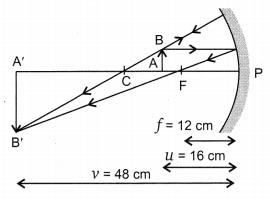Question 3.
List the sign conventions that are following in case of refraction of light through spherical lenses. Draw a diagram and apply these conventions in determining the nature and focal length of a spherical lens which forms three times magnified real image of an object placed 16 cm from the lens.   (CBSE 2012)
Sign conventions for reflection of light through spherical lens are:

1. The object is always placed to the left of the lens so that incident light moves from left to right.
2. All distances are to be measured from the optical centre of the lens.
3. The distances measured in the direction of incident light (along +ve x-axis) will be taken as positive while those measured to the left of the origin (along -ve x- axis) will be taken as negative.
4. All measurements of heights above the principal axis (along +ve y-axis) will be considered as positive while below it (along -ve y-axis) will be taken as negative.

u = – 16 cm, m = – 3
m = $$\frac{v}{u}$$ = -3
v = -3u = – 3 × (-16) = 48 cm.f = + 12 cm
Ray diagram: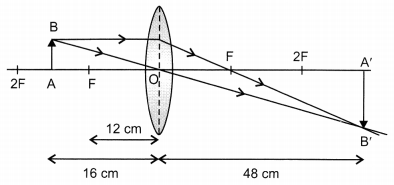Question 4.
A student has focused the image of a candle flame on a white screen using a concave mirror. The situation is as given below :   (Foreign 2015)
Length of the flame = 1.5 cm
Focal length of the mirror = 12 cm
Distance of flame from the mirror = 18 cm
If the flame is perpendicular to the principal axis of the mirror, then calculate the following :
(a) Distance of the image from the mirror
(b) Length of the image

If the distance between the mirror and the flame is reduced to 10 cm, then what would be observed on the screen? Draw ray diagram to justify your answer for this situation.
h = +1.5 cm; f = -12 cm; u = -18 cm v = ?   h’ = ?If u = -10 cm

No distinct image would be formed on the screen. In this case the image formed will be virtual (object will be within focal length).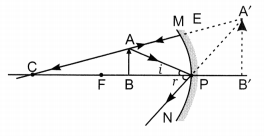Question 5.
What is meant by the power of a lens? What is its S.I. unit? Name the type of lens whose power is positive.
The image of an object formed by a lens is real, inverted and of the same size as the object. If the image is at a distance of 40 cm from the lens, what is the nature and power of the lens? Draw ray diagram to justify your answer. (Foreign 2015)

• Power of lens – It is the ability of a lens to converge or diverge light rays or it is the degree of convergence or divergence of light ray achieved by a lens (Reciprocal of focal length of the lens).
• Its S.I. unit is dioptre.
• Convex lens has positive power.

v = +40 cm; h′ = h
The lens is convex / converging.
Image is real, inverted and same sized,
∴ object is at 2F
2f = 40 cm
∴ f = 20 cm
P = $$\frac{1}{f}=\frac{100}{20 \mathrm{cm}}$$ = 5 dioptre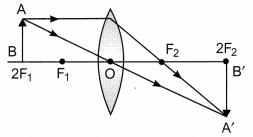Question 6.
What is meant by power of a lens? Define its S.I. unit. You have two lenses A and B of focal lengths +10 cm and -10 cm respectively. State the nature and power of each lens. Which of the two lenses will form a virtual and magnified image of an object placed 8 cm away from the lens? Draw a ray diagram to justify your answer.   (AI 2015)

• Power of lens – It is the ability of a lens to converge or diverge light rays or it is the degree of convergence or divergence of light ray achieved by a lens (Reciprocal of focal length of the lens).
• Its S.I. unit is dioptre.
1 dioptre is the power of a lens whose focal length is 1 metre.

fA = +10 cm = 0.1 m
Converging/Convex lens
PA = $$\frac{1}{f_{A}}=\frac{1}{+0.1 \mathrm{m}}$$ = +10D
fB = -10 cm = -0.1 m
Diverging/concave lens
PB = $$\frac{1}{f_{B}}=\frac{1}{-0.1 \mathrm{m}}$$ = -10D

In this case the object will be between the optical centre and principal focus of the lens. Hence the convex lens, i.e., lens A will form virtual and magnified image of the object.Question 7.
One half of a convex lens of focal length 10 cm is covered with a black paper. Can such a lens produce an image of a complete object placed at a distance of 30 cm from the lens? Draw a ray diagram to justify your answer. A 4 cm tall object is placed perpendicular to the principal axis of a convex lens of focal length 20 cm. The distance of the object from the lens is 15 cm. Find the nature, position and size of the image.   (AI 2015)

• yes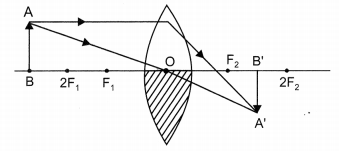(Note: image must be between F2 and 2F2)

• h = 4 cm f = +20 cm, u = -15 cm v = ?   h’ = ?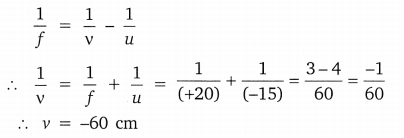Nature – Virtual, erectQuestion 8.
A convex lens can form a magnified erect as well as magnified inverted image of an object placed in front of it.” Draw ray diagram to justify this statement stating the position of the object with respect to the lens in each case. An object of height 4 cm is placed at a distance of 20 cm from a concave lens of focal length 10 cm. Use lens formula to determine the position of the image formed. (Delhi 2015)
For magnified erect image – Object is between the optical centre and principal focus of a convex lens.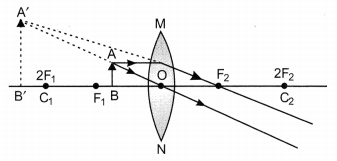For magnified inverted image-Object between F and 2F of a convex lens.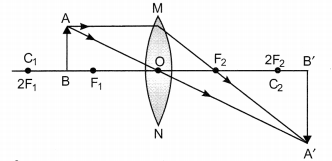Here u = -20 cm;   f = +10 cm;   v = ?Question 9.
(a) Define the following terms in the context of spherical mirrors: (Delhi 2016)
(i) Pole
(ii) Centre of curvature
(iii) Principal axis
(iv) Principal focus
(b) Draw ray diagrams to show the principal focus of a :
(i) Concave mirror
(ii) Convex mirror
(c) Consider the following diagram in which M is a mirror and P is an object and Q is its magnified image formed by the mirror.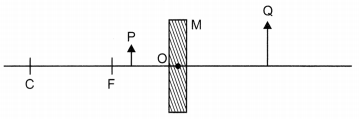State the type of the mirror M and one characteristic property of the image Q.
(a) (i) Pole – Centre of the reflecting surface of the mirror.
(ii) Centre of curvature – The centre of the hollow sphere of which the reflecting surface of mirror forms a part.
(iii) Principal axis – Straight-line passing through the pole and the centre of curvature of a spherical mirror.
(iv) Principal focus – Incident rays parallel to principal axis, after reflection, either converge to or appear to diverge from a fixed point on the principal axis called principal focus of the spherical mirror.

(b) (i)(ii)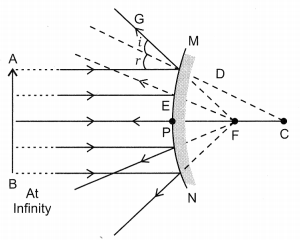(c) Concave mirror.
Image formed is virtual.

Question 10.
(a) Draw a ray diagram to show the formation of image by a convex lens when an object is placed in front of the lens between its optical centre and principal focus.
(b) In the above ray diagram mark the object-distance (u) and the image-distance (v) with their proper signs (+ve or -ve as per the new Cartesian sign convention) and state how these distances are related to the focal length (f) of the convex lens in this case.
(c) Find the power of a convex lens which forms a real, and inverted image of magnification -1 of an object placed at a distance of 20 cm from its optical centre.   (Delhi 2016)
(a)(b) Relation : $$\frac{1}{f}=\frac{1}{v}-\frac{1}{u}$$
(c) m = -1; u = -20 cm;  v = ?  f = ?
m = $$\frac{v}{u}$$ ⇒ -1 = $$\frac{v}{-20}$$ v = +20 cm
Thus object is at 2F
i.e., 2f = 20 cm
∴ f = 10 cm = 0.1 m
p = $$\frac{1}{f}=\frac{1}{0.1}$$ = +10D

Question 11.
At what distance from a concave lens of focal length 20 cm a 6 cm tall object be placed so as to obtain its image at 15 cm from the lens? Also calculate the size of the image formed. Draw a ray diagram to justify your answer for the above situation and label it.   (Foreign 2016)
f = -20 cm;  h1 = 6 cm; v = -15 cm; u = ?Lens formula: $$\frac{1}{f}=\frac{1}{v}-\frac{1}{u}$$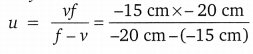= -60 cm
object at 60 cm from the lens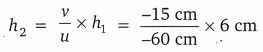= +1.5 cm diminished, erect

Question 12.
Suppose you have three concave mirrors A, B and C of focal lengths 10 cm, 15 cm and 20 cm. For each concave mirror you perform the experiment of image formation for three values of object distance of 10 cm, 20 cm and 30 cm. Giving reason answer the following:   (Foreign 2016)
(a) For the three object distances, identify the mirror/mirrors which will form an image of magnification -1.
(b) Out of the three mirrors identify the mirror which would be preferred to be used for shaving purpose/make up.
(c) For the mirror B draw ray diagram for image formation for object distances 10 cm and 20 cm.
fa = 10 cm; fb = 15 cm; fc = 20 cm
U1 = 10 cm; u2 = 20 cm; u3 = 30 cm
(a) m = -1 means u = 2f, for A → u2, for B → u3
(b) Mirror B or C – distance should be less than focal length for erect and magnified image, face is generally kept at a distance more than 10 cm.
(c)Question 13.
It is desired to obtain an erect image of an object, using concave mirror of focal length of 12 cm.   (AI 2016)
(i) What should be the range of distance of an object placed in front of the mirror?
(ii) Will the image be smaller or larger than the object? Draw ray diagram to show the formation of image in this case.
(iii) Where will the image of this object be, if it is placed 24 cm in front of the mirror? Draw ray diagram for this situation also to justify your answer.
Show the positions of pole, principal focus and the centre of curvature in the above ray diagrams.
(i) Range of distance – between 0 cm – < 12 cm
(ii) Larger than the object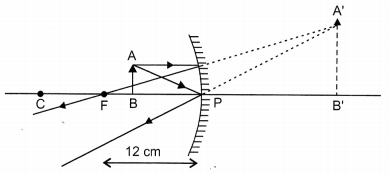(iii) Image also at 24 cm in front of the mirror.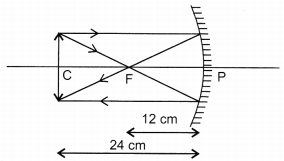Question 14.
(a) Define optical centre of a spherical lens.
(b) A divergent lens has a focal length of 20 cm. At what distance should an object of height 4 cm from the optical centre of the lens be placed so that its image is formed 10 cm away from the lens? Find the size of the image also.
(c) Draw a ray diagram to show the formation of image in above situation. (AI 2016)